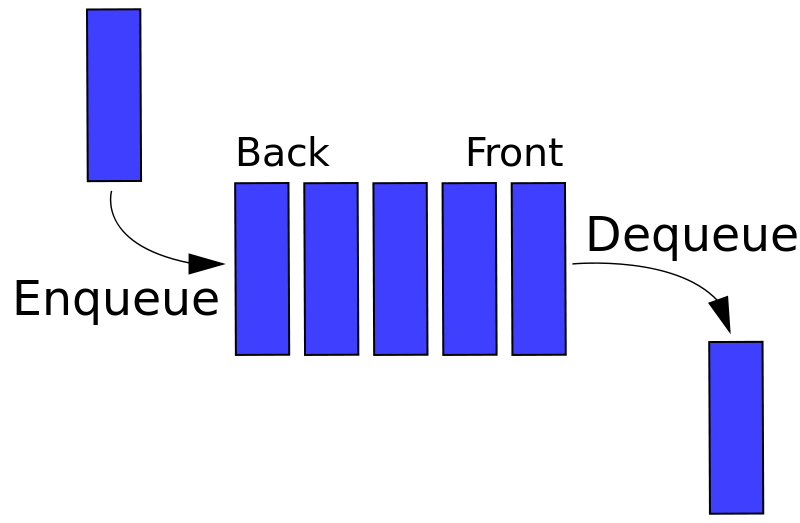# TensorFlow and Queues

Monday March 27, 2017

There are many ways to implement queue data structures, and TensorFlow has some of its own.### FIFO Queue with a list

In Python, a list can implement a first-in first-out (FIFO) queue, with slightly awkward syntax:

``````>>> my_list = []
>>> my_list.insert(0, 'a')
>>> my_list.insert(0, 'b')
>>> my_list.insert(0, 'c')
>>> my_list.pop()
'a'
>>> my_list.pop()
'b'
>>> my_list.pop()
'c'``````

A Python list is not a very efficient implementation for a first-in first-out queue, and it doesn't offer mechanisms for limiting the total length of the queue at any given time, for example. But also, queues are a common way to implement communication between threads, and not everything you might do with a list is perfectly thread-safe (though it is surprisingly close; thanks GIL).

### FIFO Queue with the Python standard library

The Python standard library's queue (`Queue` in Python 2) provides several queue options. The `queue.Queue` class implements a thread-safe FIFO queue:

``````>>> import queue
>>> my_queue = queue.Queue()
>>> my_queue.put('a')
>>> my_queue.put('b')
>>> my_queue.put('c')
>>> my_queue.get()
'a'
>>> my_queue.get()
'b'
>>> my_queue.get()
'c'``````

When making a `queue.Queue`, you can specify an integer `maxsize` argument to set a bound on how many things can be in the queue at any given time. The default is zero, which makes a queue that can store (theoretically) any number of things. And there are no restrictions on what you can put in; anything in Python can be added to one of these queues.

### Why use TensorFlow Queues?

TensorFlow also offers a number of queue options. There are a couple reasons to use TensorFlow queues over standard Python queues:

• TensorFlow queues live in TensorFlow computation graphs, with the attendant benefits of unifying things there and allowing distributed graph computation.
• TensorFlow queues offer a few more methods than standard Python queues, like `dequeue_many`, which is good for getting training batches.
• TensorFlow queues work with additional TensorFlow constructs, like the QueueRunner.
• TensorFlow offers queue variants not in the Python standard library: the PaddingFIFOQueue and RandomShuffleQueue.

### FIFO Queue with TensorFlow

Here's a standard TensorFlow FIFOQueue:

``````>>> import tensorflow as tf
>>> letter = tf.placeholder(tf.string)
>>> queue = tf.FIFOQueue(capacity=10, dtypes=[tf.string])
>>> enqueue = queue.enqueue(letter)
>>> dequeue = queue.dequeue()
>>> session = tf.Session()
>>> session.run(enqueue, feed_dict={letter: 'a'})
>>> session.run(enqueue, feed_dict={letter: 'b'})
>>> session.run(enqueue, feed_dict={letter: 'c'})
>>> session.run(dequeue)
'a'
>>> session.run(dequeue)
'b'
>>> session.run(dequeue)
'c'``````

There's a little extra code to deal with the computation graph, and the TensorFlow queue requires a `capacity` argument and a `dtypes` argument.

The `capacity` is like the `maxsize` of a regular Python queue.

The `dtypes` argument is a list of Tensorflow data types. The elements added to the queue will always be lists of tensors with the specified data types. Here, we add a single string tensor at a time to the queue.

### Comparing Python standard library and TensorFlow queue offerings

Here's a listing of queue types in the Python queue library and in TensorFlow.

### Priority Queue with the Python standard library and TensorFlow

A priority queue lets you assign a priority to each item as it is added, and the item that comes out when you next de-queue is the item with the highest priority currently in the queue. Higher priority is represented by lower numbers.

Both the standard Python queue library and TensorFlow have priority queues.

``````>>> import queue
>>> my_queue = queue.PriorityQueue()
>>> my_queue.put([4, 'a'])
>>> my_queue.put([1, 'b'])
>>> my_queue.put([2, 'c'])
>>> my_queue.get()
[1, 'b']
>>> my_queue.get()
[2, 'c']
>>> my_queue.get()
[4, 'a']``````

The TensorFlow priority queue is just like the standard Python one, but with a little more strictness on types. When adding to the queue, the first tensor provided must be `tf.int64`, the priority.

``````>>> import tensorflow as tf
>>> priority = tf.placeholder(tf.int64)
>>> letter = tf.placeholder(tf.string)
>>> queue = tf.PriorityQueue(capacity=10, types=[tf.string], shapes=[[]])
>>> enqueue = queue.enqueue([priority, letter])
>>> dequeue = queue.dequeue()
>>> session = tf.Session()
>>> session.run(enqueue, feed_dict={priority: 4, letter: 'a'})
>>> session.run(enqueue, feed_dict={priority: 1, letter: 'b'})
>>> session.run(enqueue, feed_dict={priority: 2, letter: 'c'})
>>> session.run(dequeue)
[1, 'b']
>>> session.run(dequeue)
[2, 'c']
>>> session.run(dequeue)
[4, 'a']``````

As of TensorFlow 1.0.1 at least, `tf.PriorityQueue` calls its argument `types` rather than `dtypes`, and it seems to be requiring the `shapes` argument in spite of the documentation.

### TensorFlow's `PaddingFIFOQueue`

The PaddingFIFOQueue has mini-batch model training in mind. It allows you to put in tensors of variable size, and when using `dequeue_many`, shorter ones get zero-padded to the maximum size in the batch.

``````import tensorflow as tf
numbers = tf.placeholder(tf.int64)
enqueue = queue.enqueue(numbers)
dequeue_many = queue.dequeue_many(n=3)
session = tf.Session()
session.run(enqueue, feed_dict={numbers: })
session.run(enqueue, feed_dict={numbers: [2, 3]})
session.run(enqueue, feed_dict={numbers: [3, 4, 5]})
session.run(dequeue_many)
array([[1, 0, 0],
[2, 3, 0],
[3, 4, 5]])``````

### TensorFlow's `RandomShuffleQueue`

The RandomShuffleQueue also has batching in mind. It draws randomly from items currently in the queue.

To prevent pulling out items as they go in (not at all randomly) the `RandomShuffleQueue` has an argument `min_after_dequeue` which ensures that there are at least that many (plus the number being drawn) to randomly draw from. This requirement is dropped after the queue eventually has `.close()` called on it, so that all items can be drawn.

``````>>> import tensorflow as tf
>>> letter = tf.placeholder(tf.string)
>>> queue = tf.RandomShuffleQueue(capacity=10, dtypes=[tf.string],
...                               min_after_dequeue=2)
>>> enqueue = queue.enqueue(letter)
>>> dequeue = queue.dequeue()
>>> session = tf.Session()
>>> session.run(enqueue, feed_dict={letter: 'a'})
>>> session.run(enqueue, feed_dict={letter: 'b'})
>>> session.run(enqueue, feed_dict={letter: 'c'})
>>> session.run(dequeue)
'b'  # or 'a', or 'c'``````

### Queues inside TensorFlow

Some parts of TensorFlow automatically create and use queues. For example, tf.train.string_input_producer (among others in input.py) makes a queue, and a QueueRunner, and sets up a TensorBoard summary op.

I'm working on Building TensorFlow systems from components, a workshop at OSCON 2017.